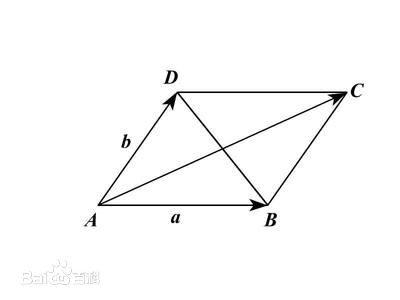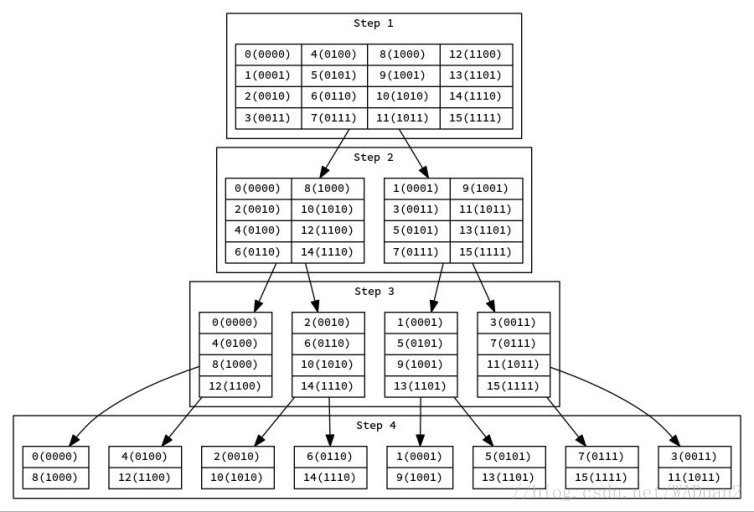# 快速傅里叶变换（FFT）学习笔记

## 前言

FFT （ Fast Fourier Transformation ）是离散傅氏变换（ DFT ）的快速算法。即为快速傅氏变换。它是根据离散傅氏变换的奇、偶、虚、实等特性，对离散傅立叶变换的算法进行改进获得的。这里仅讨论 FFT 在 OI 中的应用。

## 复数

### 平行四边形定则## 单位根

### 性质

• $\omega _{n} ^{k} = \cos{(k \frac{2\pi}{n})} + i \sin{(k \frac{2\pi}{n})}$ ，即欧拉公式

• $\omega {2n} ^{2k} = \omega {n} ^{k}$

证明：

• $\omega {n} ^{k + \frac{n}{2}} = -\omega {n} ^{k}$

证明：

• $\omega {n} ^{0} = \omega {n} ^{n} = 1$

## 非递归 FFT## 后记

https://www.cnblogs.com/zwfymqz/p/8244902.html

0%